# R语言：ggplot2精细化绘图——以实用商业化图表绘图为例

1、画图前的准备：自定义ggplot2格式刷
2、画图前的准备：数据塑形利器dplyr / tidyr介绍
3、常用的商业用图：

1）简单柱形图+文本（单一变量）
2）分面柱形图（facet_wrap/facet_grid)
3）簇型柱形图(position=”dodge”)
4）堆积柱形图(需要先添加百分比，再对百分比的变量做柱形图)
5）饼图、极坐标图
6）多重线性图

#### 1、画图前的准备：自定义ggplot2格式刷

library(ggplot2)
library(dplyr)
library(ColorBrewer)
library(tidyr)
library(grid)
#载入格式刷
######

#定义好字体
windowsFonts(CA=windowsFont("Calibri"))

 1 #定义好字体
2 windowsFonts(CA=windowsFont("Calibri"))
3 #事先定制好要加图形的形状、颜色、主题等
4 #定制主题，要求背景全白，没有边框。然后所有的字体都是某某颜色
5 #定制主题，要求背景全白，没有边框。然后所有的字体都是某某颜色
6 mytheme<-theme_bw()+theme(legend.position="top",
7                           panel.border=element_blank(),
8                           panel.grid.major=element_line(linetype="dashed"),
9                           panel.grid.minor=element_blank(),
10                           plot.title=element_text(size=15,
11                                                   colour="#003087",
12                                                   family="CA"),
13                           legend.text=element_text(size=9,colour="#003087",
14                                                    family="CA"),
15                           legend.key=element_blank(),
16                           axis.text=element_text(size=10,colour="#003087",
17                                                  family="CA"),
18                           strip.text=element_text(size=12,colour="#EF0808",
19                                                   family="CA"),
20                           strip.background=element_blank()
21
22                         )
23 pie_theme=mytheme+theme(axis.text=element_blank(),
24                         axis.ticks=element_blank(),
25                         axis.title=element_blank(),
26                         panel.grid.major=element_blank())
27 #定制线的大小
28 myline_blue<-geom_line(colour="#085A9C",size=2)
29 myline_red<-geom_line(colour="#EF0808",size=2)
30 myarea=geom_area(colour=NA,fill="#003087",alpha=.2)
31 mypoint=geom_point(size=3,shape=21,colour="#003087",fill="white")
32 mybar=geom_bar(fill="#0C8DC4",stat="identity")
33 #然后是配色，考虑到样本的多样性，可以事先设定颜色，如3种颜色或7种颜色的组合
34 mycolour_3<-scale_fill_manual(values=c("#085A9C","#EF0808","#526373"))
35 mycolour_7<-scale_fill_manual(values=c("#085A9C","#EF0808","#526373",
37 mycolour_line_7<-scale_color_manual(values=c("#085A9C","#EF0808","#526373",
38                                              "#0C8DC4","#FF9418","#219431","#9C52AD"))

1）先生成一个简单的图表：

#未使用格式刷
p<-ggplot(iris,aes(x=species,y=sepal_length)) +geom_bar(stat="identity")+ ggtitle("sepal_length by species")
p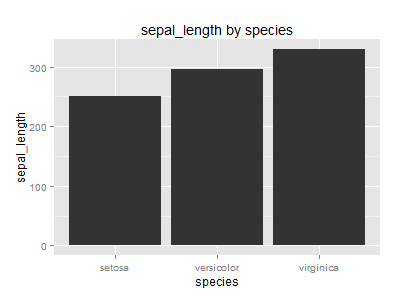p+mytheme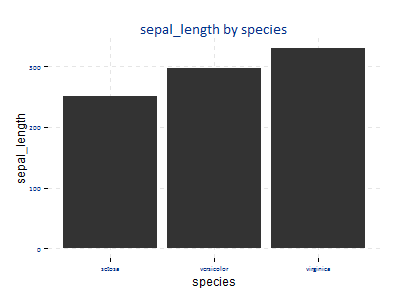ggplot(iris,aes(x=species,y=sepal_length)) +ggtitle("sepal_length by species")+mybar+mytheme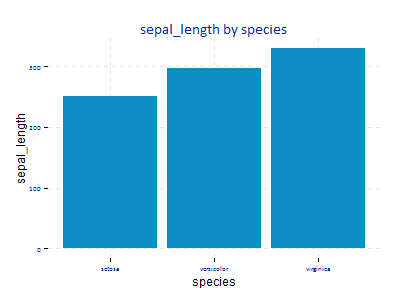#### 2、画图前的准备：数据塑形利器dplyr / tidyr

install.packages("swirl")
library(swirl)
#安装getting and cleaning data相关的课程教学包
install_from_swirl("Getting and Cleaning Data")
swirl()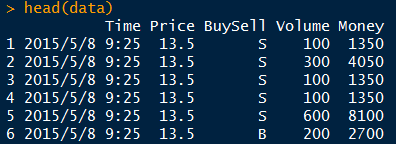data #刚刚演示的那些数据,在预测者网可以下载
data %>% group_by(Price,BuySell) %>% summarize(Money=sum(Money,na.rm=TRUE)) %>% spread(BuySell,Money)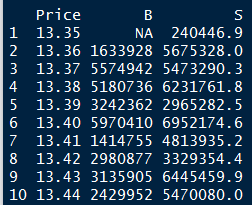#### 3、常用的商业用图

1、简单柱形图+文本（单一变量）
2、分面柱形图（facet_wrap/facet_grid)
3、簇型柱形图(position=”dodge”)
4、堆积柱形图(需要先添加百分比，再对百分比的变量做柱形图)
5、饼图、极坐标图
6、多重线性图

ggplot2最大的局限是，它基本不支持双坐标图和饼图。即使能做这些图形，也要很多设置，做起来非常繁琐。

It’s not possible in ggplot2 because I believe plots with separate y scales (not y-scales that are transformations of each other) are fundamentally flawed.

mytitle="演示：以diamond为例"

1）简单柱形图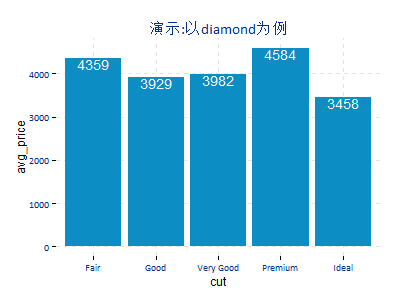data1<-diamonds %>% group_by(cut) %>% summarize(avg_price=mean(price))

mytitle+mybar+mytheme+
geom_text(aes(label=round(avg_price)),vjust=1,colour="white")

2）带分类的柱形图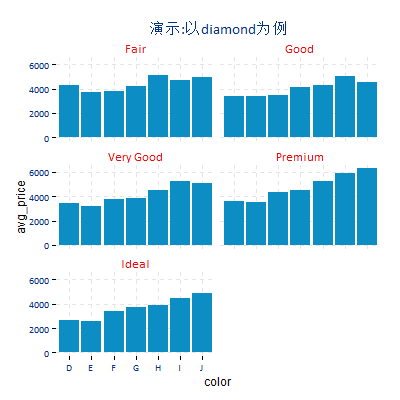#dplyr处理数据
data2<-diamonds %>% group_by(cut,color) %>% summarize(avg_price=mean(price))
#画图，套用设定好的绘图元素
ggplot(data2,aes(x=color,y=avg_price))+facet_wrap(~cut,ncol = 2)+
mytitle+mybar+mytheme
#在facet_wrap里面，如果加上scales="free"的话，坐标就不一样了。

3）簇型图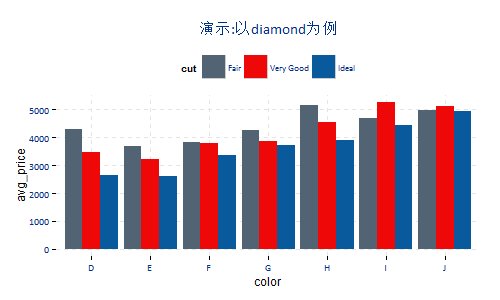data3<-diamonds %>% filter(cut %in% c("Fair","Very Good","Ideal")) %>%
group_by(cut,color) %>% summarize(avg_price=mean(price))
#簇状图

geom_bar(stat="identity",position="dodge")+
mytheme+mytitle+mycolour_3

data3$cut<-factor(data3$cut,levels=c("Very Good","Ideal","Fair"))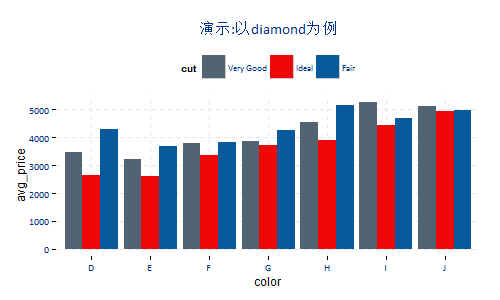4）百分比堆积图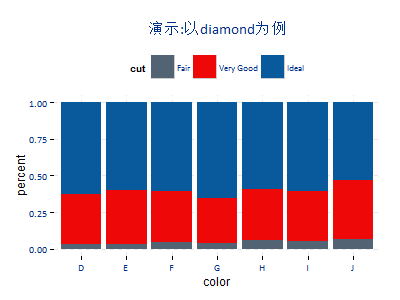data4<-diamonds %>% filter(cut %in% c("Fair","Very Good","Ideal")) %>%
count(color,cut) %>%
mutate(percent=n/sum(n))

geom_bar(stat="identity")+mytheme+mytitle+mycolour_3

5）饼图以及极坐标图

1）不指定x轴，直接用geom_bar生成y轴，然后fill=分类颜色，coord_polar直接投影y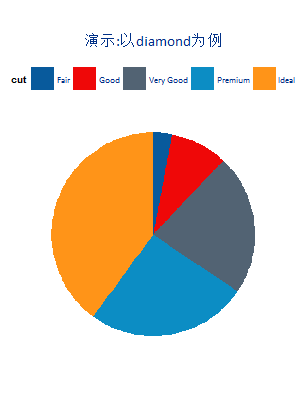data5<-diamonds %>% count(cut) %>%
mutate(percent=n/sum(n))
ggplot(data5,aes(x=factor(1),y=percent,fill=cut))+geom_bar(stat="identity",width=3)+mycolour_7+
coord_polar("y")+pie_theme+mytitle

2）指定x轴，x轴同时也是颜色(fill)，先画柱形图，再转化为圆形。坏处是公式相对比较繁琐一些。

ggplot(data5,aes(x=cut,y=percent,fill=cut))+
geom_bar(stat="identity",width=3)+
mycolour_7+coord_polar("x")+pie_theme+mytitle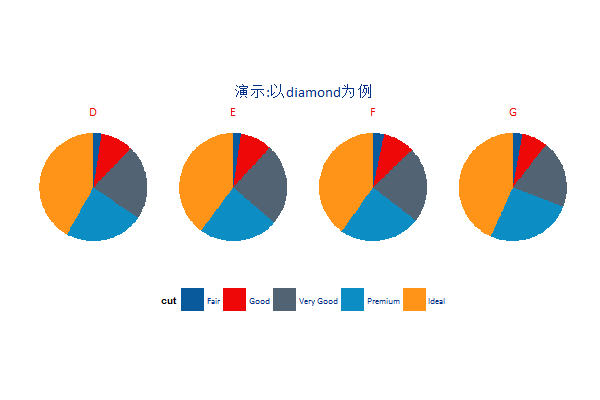data5_1<-data5 %>% filter(color %in% c("D","E","F","G"))
ggplot(data5_1,aes(x=factor(1),y=percent,fill=cut))+geom_bar(stat="identity",width=3)+mycolour_7+
coord_polar("y")+pie_theme+facet_wrap(~color,ncol = 4)+
theme(legend.position="bottom")+mytitle

6、折线图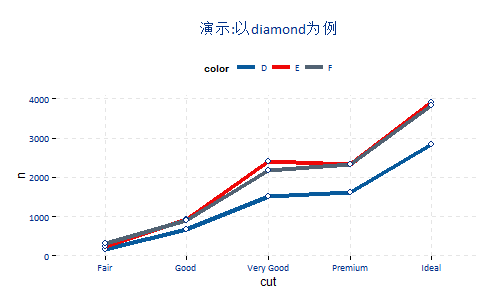data6<-diamonds %>% count(color,cut) %>% filter(color %in% c("D","E","F"))%>%
mutate(percent=n/sum(n))
ggplot(data6,aes(x=cut,y=n,group=color,colour=color))+geom_line(size=1.5)+mypoint+
mycolour_line_7+mytheme+mytitle

##### 参考资料：

1、刘万祥老师的商业图表系列
2、R可视化技术
3、ggplot2:数据分析与图表技术
4、万能的stackoverflow
5、ggplot2
6、配色相关：

html两色搭配大全，以及html按风格分的配色盘

posted @ 2015-06-10 23:34  尾巴AR  阅读(17172)  评论(2编辑  收藏